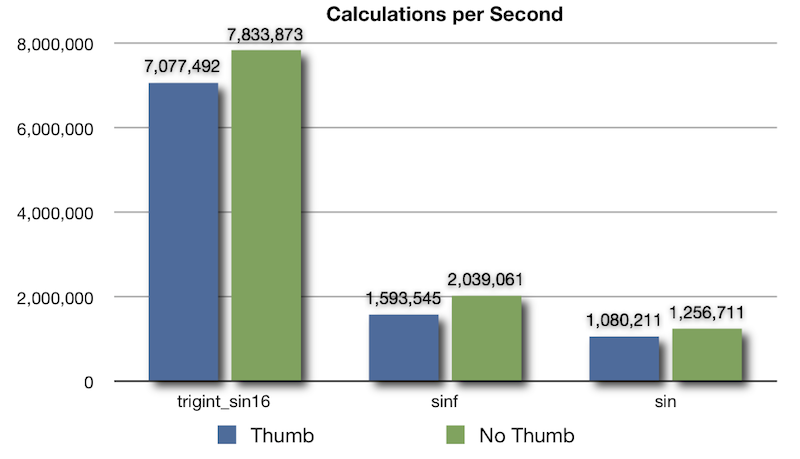# Performance of trigint_sin16 on iOS

iOS devices run on ARM processors.

While the ARM processor is capable of performing floating point calculations in hardware, it is still faster to execute integer-based calculations. Also, iOS applications are compiled in Thumb mode, by default. While thumb mode can reduce the code size by up to 35%, Apple recommends applications that make extensive use of floating point math compile without Thumb mode.

Using the trigint library on iOS not only provides much faster trigonometry calculations, but it allows applications to be compiled in Thumb mode, since no floating point is used.

The following graph shows the performance gains on a 1st generation iPod Touch between trigint_sin16() and the standard library sinf() and sin() functions:The sinf() and sin() functions were used to calcuate an integer value between -32,767 and +32,767 as such:

``` int16_t sinf_value = roundf(32767.0f * sinf(angle));
int16_t sin_value = round(32767.0 * sin(angle));
```

trigint_sin16() is about 4.4 times faster than sinf() and 6.7 times faster than sin() in Thumb mode. Without Thumb mode, the gap closes a bit to 3.8 times faster and 6.2 times faster, respectively.

Note that the floating point values are converted to an integer using the standard roundf() and round() functions plus a cast. If you don't need the accuracy, you can skip the roundf() or round() and just use a cast. This increases performace by about 15% (in Thumb mode), but accuracy is lost as the floating point to integer conversion just truncates the fractional part. trigint_sin16() does rounding, which is why roundf() and round() were used for the benchmarks.